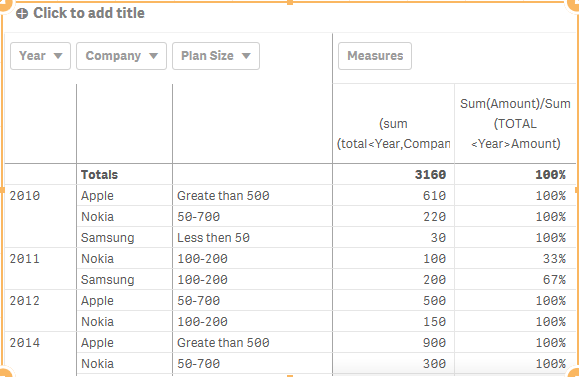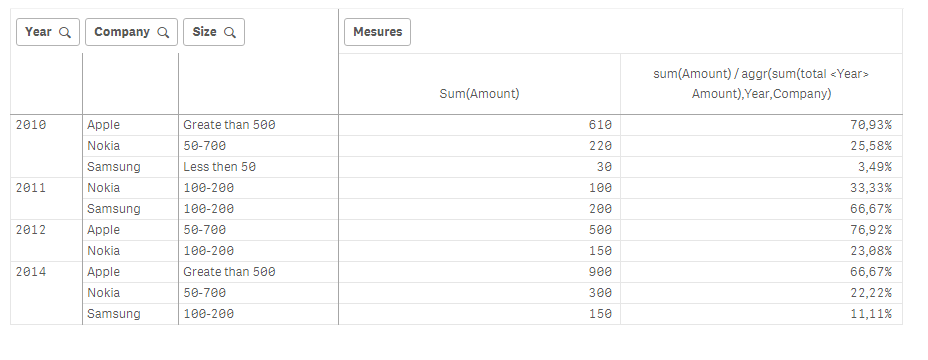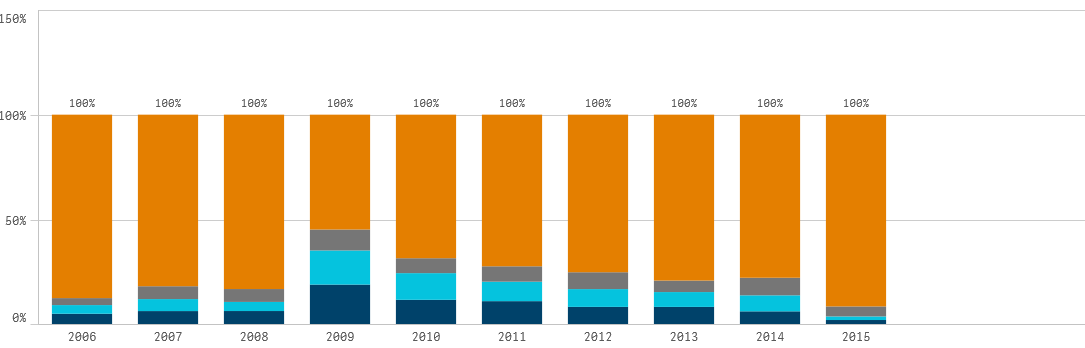# New to Qlik Sense

Discussion board where members can get started with Qlik Sense.

Highlighted
Contributor II

## Calculate Percentage of Multidimensional columns

Hello Community,

I want to create a Percentages based on the range of Amount by Year and Company. Please find the details below. I tried a couple of approaches but I am not able to achieve the expected result.

Actual Table:

 Year Company Amount 2010 Apple 10 2010 Nokia 20 2010 Samsung 30 2010 Apple 100 2010 Nokia 200 2010 Apple 500 2011 Nokia 100 2011 Samsung 200 2012 Apple 500 2012 Nokia 150 2014 Apple 600 2014 Nokia 100 2014 Samsung 150 2014 Apple 300 2014 Nokia 200

Result Table: This is giving me the percentages of all the years.

 Year Company Plan Size Total Amount Percentages Totals 3160 100% 2010 Apple Greate than 500 610 19% Nokia 50-700 220 7% Samsung Less then 50 30 1% 2011 Nokia 100-200 100 3% Samsung 100-200 200 6% 2012 Apple 50-700 500 16% Nokia 100-200 150 5% 2014 Apple Greate than 500 900 28% Nokia 50-700 300 9% Samsung 100-200 150 5%

Dimension Expression:

Plan Size:

if(aggr(sum(Amount),Year,Company)<50,'Less then 50',

if(aggr(sum(Amount),Year,Company)>=100 and aggr(sum(Amount),Year,Company)<=200 ,'100-200',

if(aggr(sum(Amount),Year,Company)>500,'Greate than 500',

if(aggr(sum(Amount),Year,Company)>50 and aggr(sum(Amount),Year,Company)<700 ,'50-700',

))))

Measure Expression:

Total Amount:

(sum(total<Year,Company>Amount))

Percentage Amount:

sum(Amount)/(sum(total{\$<Year,Company>}Amount))

But the Expected Result I want is to show the 100% split per each year.

Expected Result:

 Year Company Plan Size Total Amount Percentages 2010 Apple Greate than 500 610 71% 2010 Nokia 50-700 220 26% 2010 Samsung Less than 50 30 3% Total 860 100% 2011 Nokia 100-200 100 33% 2011 Samsung 100-200 200 67% Total 300 100% 2012 Apple 50-700 500 77% 2012 Nokia 100-200 150 23% Total 650 100% 2014 Apple Greate than 500 900 67% 2014 Nokia 50-700 300 22% 2014 Samsung 100-200 150 11% Total 1350 100%

Also, I will convert this table to 100% Stacked bar color by PLAN SIZE. So that I can see how much percentage of plan size for each year.

-Vidya

1 Solution

Accepted Solutions
Contributor II

## Re: Calculate Percentage of Multidimensional columns

So, With all the respect and information provided by the gentlemen in this thread. Both the expressions will give the right output.

Measure Expressions:

Sum(Amount)/Aggr(nodistinct sum(Amount),Year)

OR

sum(Amount) / aggr(sum(total <Year> Amount),Year,Company)

Since my report consuming more than 10 Million records of data. I have to test which is the better one in terms of

performance.

Thanks,

-Vidya

16 Replies
Contributor II

## Re: Calculate Percentage of Multidimensional columns

Hi All,

I was able to find the solution. All I need to change the percentage expression. Which is.

sum(Amount)/aggr(nodistinct sum(Amount),Year).

However, I have to convert this result set into a 100% stacked bar.

Thanks,

-VidyaMVP

## Re: Calculate Percentage of Multidimensional columns

Better yet, use this

Sum(Amount)/Sum(TOTAL <Year>Amount)

Contributor II

## Re: Calculate Percentage of Multidimensional columns

I tried this expression but I am getting the wrong Percentage split.Thanks,

-VidyaMVP

## Re: Calculate Percentage of Multidimensional columns

Seems like something to do with the calculated dimension.... Not sure why it creates an issuePartner

## Re: Calculate Percentage of Multidimensional columns

How come?MVP

## Re: Calculate Percentage of Multidimensional columns

add the calculated dimension and you will see what he means

Contributor II

## Re: Calculate Percentage of Multidimensional columns

Yes. If you add the calculated dimension the results will vary.

if(aggr(sum(Amount),Year,Company)<50,'Less then 50',

if(aggr(sum(Amount),Year,Company)>=100 and aggr(sum(Amount),Year,Company)<=200 ,'100-200',

if(aggr(sum(Amount),Year,Company)>500,'Greate than 500',

if(aggr(sum(Amount),Year,Company)>50 and aggr(sum(Amount),Year,Company)<700 ,'50-700',

))))

Thanks,

-VidyaPartner

## Re: Calculate Percentage of Multidimensional columns

Ah I see !

change ur measure to :

sum(Amount) / aggr(sum(total <Year> Amount),Year,Company)

result:Contributor II

## Re: Calculate Percentage of Multidimensional columns

Hi,

After converting the Pivot table to a 100% stacked bar chart. I got it what I want.. There might be a better solution than this. But as of now, this solution helped me in fixing my issue.Thanks,

-Vidya